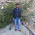### Maths tricks and short cuts for exams Bank PO, SSC, Clerical, Insurance, CSAT, others

MATHEMATICS FUNDAE FOR CLERICAL, BANK PO, SSC EXAMS AND OTHERS EXAMS

 To find the number of factors of a given number, express the number as a product of powers of prime numbers. Take the example of 576.          576 = 64*9 = 26 x 32 It is like 20, 21, 22, 23, 24, 25, 26   X   30, 31, 32 To get No. Of factors what is there is that you take any thing from 2's power multiply by anthing from 3's power, that will divide your initial number because it has that many  number of 2's and 3's . Right. So how many combination are possible ? On left are 7 and right are 3 so net will be 7 x 3 =21 factors. In other words just remember After finding that 26 x 32 step, just do (6+1) x (2+1) = 7 x 3 =21 factors.
Note - If question ask you not to count 1 & 576 as factors, then subtract 2 from answer means 21-2=19. Otherwise don't subtract unnecessarily.

The sum of first n natural numbers = n (n+1)/2

Eg The sum of 1+2+3+……..+10+11+12 = ??

Here n = 12 so Sum     = 12 ( 12+1 ) / 2
= 12 * 13 /2 = 6 * 13 = 78

The sum of squares of first n natural numbers is   n (n+1)(2n+1)/6

Eg the sum of 12 + 22 + 32 + 42 + ………. +122 =??

= 12 (12+1) (2*12 + 1) /6
= 12*13*25 /6 = 650

The sum of first n even numbers= n (n+1)

Eg The sum of 2+4+6+8+10+…………. +40 = ??
Here n ≠40. N is the no of terms. So number of terms here is 20, the value of 20th term is 40 indeed. So with n = 20

 Sum = 20 (20+1) =20*21 =420 The sum of first n odd numbers= n2 Eg The sum of 1+3+5+7+9+11+…………..+21 Here n≠21. Here number of terms are 11 so n=11.                         Sum = 112 = 121

To find the squares of numbers near numbers of which squares are known
For eg you might know squares of 20,30,40,.. Very easily.
So
To find square of 41 which is near to 40, Add 40+41 to 1600 =1681

To find 592 , which is near to 60, Subtract 602  - (60+59)  =3600-119 = 3481

Add when the number is more and subtract when the number is less.
Keep Yourself updated with job openings in different sectors.
------------------------------------------------------------------------------------------------------------------- Keywords - Maths Shortcuts, Maths Tricks, Bank PO and Clerical Maths tricks and shortcuts, quick calculations of maths, -------------------------------------------------------------------------------------------------------------------

1.This comment has been removed by a blog administrator.

2.योग इकाई हो गुरु दहाई,
एक समान हो गिनती बाँयीं ।
एक दहाई में जोड़ो एक,
गुणा करो और दे दो फेंक ॥

उदाहरण 1- 34 को 36 से गुणा करने के लिए किसी एक दहाई में एक जोड़कर यानी 3+1 = 4x3=12 व इकाई का गुणा इकाई से गुणा यानी 4x6=24 होगा। दोनों संख्यायें बराबर लिखने पर उत्तर प्राप्त हो जायेगा: 1224 (देखें चित्र)
उदाहरण 2- 413 X 487
413 X 487
= 4 x 5 / 13 x 87 (एक दहाई में एक जोड़ा )
= 20 /1131 (दहाई X दहाई/ इकाई X इकाई)

World’s No. 1 Brain Training and Memory Book
 World’s only colored book of Memory and Mental Math.
 World’s only Brain book having more than 200 colored illustrations.
 World’s only book which trains logic and verbal memory.
 World’s only book of Guru Ganit, simpler from Vedic math.
 N L Shraman is the only Hindi and English Mnemonist in the World. http://en.wikipedia.org/wiki/Mnemonist.
 World’s only book which trains basics of 51 subjects to a child of 4 years.
 World’s School teaches what to read, this book teaches how to read.
 World’s only book an alternative to tuitions and teacher of teachers.
 World’s only book for all ages: Students, Teachers, Working, Old Young.
 Browse pages on : http://books.google.co.in/books?id=raRVq78byAUC
 World’s only book which improves grades in school immediately.
 World’s only book which improves memory instantly and removes panic and fear in Exam.
परीक्षा का एक (1) नम्बर आपकी जिन्दगी बदल सकता है पर रु 1001 नहीं।

3.Nice and easiy trick

www.computertreat.com

4.